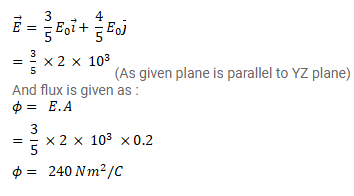# The electric field in a region is given byQuestion:

The electric field in a region is given by

$\vec{E}=\frac{3}{5} E_{0} \vec{\imath}+\frac{4}{5} E_{0} \vec{\jmath}$ with $E_{0}=2.0 \times 10^{3} \mathrm{~N} / \mathrm{C}$

Find the flux of this field through a rectangular surface of area $0.2 \mathrm{~m}^{2}$ parallel to the $\mathrm{Y}-\mathrm{Z}$ plane.

Solution: